UPSC  >  Overview: Time & Work

# Overview: Time & Work - Notes | Study CSAT Preparation for UPSC CSE - UPSC

 Table of contentsConceptsBasic FormulaSome Important FormulasPractice Questions1 Crore+ students have signed up on EduRev. Have you?

Time and Work is an important topic in the Quantitative Aptitude section of the CAT exam. The questions related to time and work are asked every year and holds a good weightage as the various applications of time and work.• Time and work deal with the time taken by an individual or a group of individuals to complete a piece of work and the efficiency of the work done by each of them.

The problems on Time and Work fall into two categories:

• To find the time required to complete the assigned job.
• To find the work done in a given time period.

Concepts

• Work is defined as something which has an effect or outcome; often the one desired or expected. The basic concept of Time and Work is similar to that across all Arithmetic topics, i.e. the concept of Proportionality.

• Efficiency is inversely proportional to the Time taken when the amount of work done is constant.
i.e. Efficiency 1/Time taken

Basic Formula

• If a man can complete a work in 'n' days, then his one day's work = 1/n, and this one day's work in terms of percentage is called Capacity
If a man can complete 1/n work in one day, he can complete the whole work in n days.
• Here, we assume that total work is one (unit); the number of days required to complete the given work would be equivalent to the reciprocal of the day's work.

A man can complete his work in n days.

His one day's work = 1/n

His percentage capacity = (1/n) x 100These type of problems can be solved through two methods:

1. Unitary method
2. Percentage capacity

The unitary method is obsolete due to its difficult calculations.

Note:

• More men less days and conversely more days less men.
• More men more work and conversely more work more men.
• More days more work and conversely more work more days.

Some Important Formulas

Type - 1

• If M1 men can do W1 work in D1 days and M2 men can do W2 work in D2 days, then: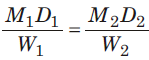• If we include working hours T1 and T2 for two groups, then: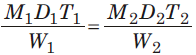• If M1 men of E1 efficiency can do W1 work in D1 days with T1 working hours/day and M2 men of E2 efficiency can do W2 work in D2 days with T2 working hours/day, then: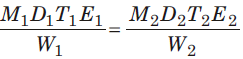Example.1. A contractor adopts a work to complete it in 100 days. He employed 100 workers. But after 50 days it was found that only 1/3rd of the work has completed, How many more workers he should employ to complete the work in time.
(a) 100
(b) 125
(c) 150
(d) 175

• 100 men in 50 days can do 1/3rd of the work.
• No. of men required to complete 2/3rd of the work in 50 days will be: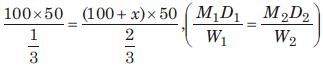• Hence, x = 100

Question for Overview: Time & Work
Try yourself:Ram is twice as efficient as Sunita and can finish a piece of work in 25 days less than Sunita. Sunita can finish this work in how many days?

Example 2: If 25 men can do a piece of work in 36 days working 10 hours a day, then how many men are required to complete the work working 6 hours a day in 20 days?
(a) 65
(b)
75
(c)
84
(d)
92

• M1 x D1 x H1 = M2 x D2 x H2
⇒ 25 x 36 x 10 = M2 x 20 x 6
⇒ M2 = 75 persons
• Alternatively: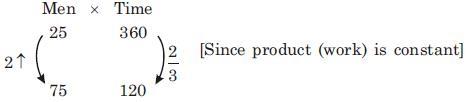• By percentage change graphic, when time is decreased by 2/3 ( i.e., 66.66%), the number of men is increased by 2 times (i.e., 200%)

Example 3: A contractor employed a certain number of workers to finish construction of a road in a certain scheduled time. Sometime later, when a part of work had been completed, he realised that the work would get delayed by three-fourth of the scheduled time, so he at once doubled the number of workers and thus he managed to finish the road on the scheduled time. How much work had been completed, before increasing the number of workers?

(a) 10%
(b)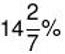(c) 12 %
(d) 15 %

• Let he initially employed x workers which works for D days and he estimated 100 days for the whole work and then he doubled the workers for (100 - D) days.
• D * x + (100 - D) * 2x = 175x
D = 25 days
• Note: 175 = 100 + (3/4) x 100, Since required number of days are 75% i.e (3/4) more than the estimated number of days.
• Now, the work done in 25 days = 25x
Total work =175x
• ∴ Work done before increasing the number of workers =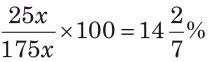Type - 2

• If A can do a piece of work in x days and B can do it in y days then A and B working together will do the same work in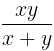days.

Example 4: A and B can do a piece of work in 12 days, B and C in 15 days C and A in 20 days. How long would each take separately to do the same work?
(a)
A = 20 days, B = 30 days, C = 50 days
(b)
A = 30 days, B = 20 days, C = 60 days
(c)
A = 20 days, B = 20 days, C = 50 days
(d) A = 30 days, B = 40 days, C = 30 days

• A + B can do in 12 days, B + C can do in 15 days, and A+ C can do in 20 days.
• 2(A+ B + C) =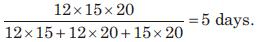• ∴ A+ B + C can do the work in 5 x 2 =10 days. (less men more days)
• Now,
⇒ A can do the work in =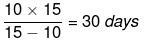[As, A =(A+ B + C) - (B + C)]
⇒ B can do the work in =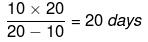[As, B = (A+ B + C) - (A+ C)]
⇒ C can do the work in =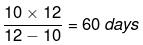Question for Overview: Time & Work
Try yourself:Railneer is packaged in a water bottling plant, with the help of two machines M1 and M2. M1 and M2 produce 400 and 600 bottles per minute respectively. One day's production can be processed by M1 operating alone for 9 hours, by M2 operating alone for 6 hours or by both M1 and M2 operating simultaneously for 3 hours and 36 minutes. If one day's production in processed by M1 operating alone for 1/3 of the time and M1 and M2 simultaneously operating for 2/3 of the time, then in how many hours total production of one day will be completed?

Type - 3

• If A, B, and C do a work in x, y, and z days respectively then all three working together can finish the work in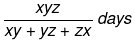Example 5: A can complete a work in 10 days B in 20 days and C in 25 days. If they work together in how many days they can complete the work.
(a)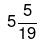(b)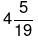(c)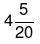(d)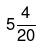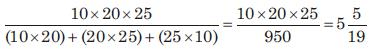Type - 4

• If A and B together can do a work in x days and A alone can do it in y days then B alone can do it in xy/(y-x) days.

Example 6: The rate at which tap M fills a tank is 60% more than that of tap N. If both the taps are opened simultaneously, they take 50 hours to fill the tank. The time taken by N alone to fill the tank is (in hours):
(a) 90 hours
(b)
110 hours
(c)
130 hours
(d)
150 hours

• If the rate at which Tap N fills the tank is 10 units per hour, the rate of Tap M would be 16 units per hour.
• Hence,
⇒ the capacity of the tank would be 26 x 50 =1300.
⇒ Time taken by Tap N alone would be 1300 /10 =130 hours

Question for Overview: Time & Work
Try yourself:25 men and 15 women can complete a piece of work in 12 days. All of them start working together and after working for 8 days the women stopped working. 25 men completed the remaining work in 6 days. How many days will it take for completing the entire job if only 15 women are put on job.

Type - 5

• When the value of one quantity increases with respect to decrease in other or vice-versa, then they are said to be inversely proportional. It means that the two quantities behave opposite in nature.
• Efficiency is inversely proportional to the number of days (D) taken to complete the work. Means,
E ∝ 1/D or E  = K/D Where K is constant
∴ ED = constant
Similarly, E1D1 = E2D2
• These product methods are limited to the constant work, if the amount of work gets changed, then it does not work, then we have to take help from the Unitary method.
• In the unitary method, the value of a unit quantity is calculated first to calculate the value of other units. It has two types of variations:
(i) Direct Variation: In direct variation, increase or decrease in one quantity will cause an increase or decrease in another quantity. For instance, an increase in the number of goods will cost more price.
(ii) Inverse Variation: It is the inverse of direct variation. If we increase a quantity, then the value of another quantity gets decrease.
• When more than one man works on a particular work, the rate of work is calculated as the strength of workers working at a particular time. So, the amount of work is defined in terms of men days or man-hours or man-days-hours.

Example 7: A takes 16 days to finish a job alone, while B takes 8 days to finish the same job. What is the ratio of their efficiency and who is less efficient?
(a) 1 : 2
(b) 1 : 3
(c) 2 : 3
(d) 3 : 4

• Since A takes more time than B to finish the same job hence A is less efficient.
Efficiency of A = 100/16 = 6.25 %
Efficiency of B = 100/8 = 12.5 %
• Ratio of efficiency of A and B,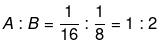Hence, B is twice efficient as A.

Note:

•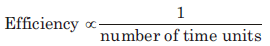∴ Efficiency * Time taken = Constant work
•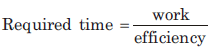Whole work is always considered as 1, in terms of fraction and 100%, in terms of percentage.
• In general, the number of days or hours = (100 / Efficiency)

Question for Overview: Time & Work
Try yourself:PYQ (CAT 2019): At their usual efficiency levels, A and B together finish a task in 12 days. If A had worked half as efficiently as she usually does, and B had worked thrice as efficiently as he usually does, the task would have been completed in 9 days. How many days would A take to finish the task if she works alone at her usual efficiency?

Practice Questions

Q.1. A can complete a work in 10 days, B in 12 days and C in 15 days. All of them began the work together, but A had to leave the work after 2 days of the start and B 3 days before the completion of the work. How long did the work last?

(a) 5
(b) 6
(c) 7
(d) 8

• See the diagram and then interpret the language of the question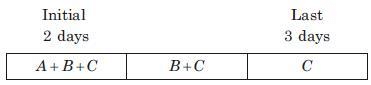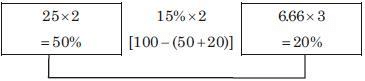• Since initially for 2 days all of them A,B and C work together so they complete he 50% work and for the last 3 days only C works which is equal to 20% work.
• Thus, the remaining work = 30%[100 - (50 + 20)]
• This 30% work was done by B and C in 2 days = (30/15)
• Note:
Efficiency of A =10%
Efficiency of B = 8.33%
Efficiency of C = 6.66%
• So, the total number of required days = 2 + 2 + 3 = 7 days.

Q.2. Arun and Satyam can complete a work individually in 12 days and 15 days respectively. Arun does work only on Monday, Wednesday and Friday while Satyam does the work on Tuesday, Thursday and Saturday. Sunday is always off. But Arun and Satyam both work with half of their efficiencies on Friday and Saturday respectively. If Arun started the work on 1st January which falls on Monday followed by Satyam on the next day and so on (i.e., they work collectively on alternate days), then on which day work will be completed?

(a) Tuesday
(b) Thursday
(c) Friday
(d) Saturday

• {This pattern continued for total 2 weeks only till 75% work got completed.}
• Thus in 2 weeks they will complete 75% work.
• Now 15% of the remaining (25% of the work) will be done in the third week on Monday and Tuesday. Again 10% work remained undone. Out of this 8.33 work will be done by Arun on Wednesday and remaining 1.66% work will be completed on Thursday by Satyam.
• Hence the work will be completed on Thursday.

Q.3. Boston, Churchill and David are three workers, employed by a contractor. They completed the whole work in 10 days. Initially, all of them worked together, but the last 60% of the work was completed by only Churchill and David together. Boston worked with Churchill and David only for initial two days then he left the work due to his poor health. Also Churchill takes 20% less time to finish the work alone then that of David working alone. If they were paid ₹ 3000 for the entire work, then what is the share of least efficient person?

(a) 33.33%
(b) 33%
(c) 33%
(d) 33%

• From the above diagram it is clear that efficiency of C and D is 7.5%, since C and D complete 60% work in 8 days and efficiency of B,C and D is 20%. It means efficiency of B alone is 12.5%= (20 – 7.5).
• ∴ Efficiency of C and Efficiency of D. Thus D is the least efficient person.
• Now share of work done by David (D) = 3.33% x 10 = 33.33%
• Hence, his share of amount = 33.33% of  ₹ 3000 is ₹ 1000

Q.4. In the ancient city of Portheus, the emperor has installed an overhead tank that is filled by two pumps X and Y. X can fill the tank in 12 hours while Y can fill the tank in 15 hours. There is a pipe Z which can empty the tank in 10 hours. Both the pumps are opened simultaneously. The supervisor of the tank, before going out on a work, asks his assistant to open Z when the tank is exactly 40% filled so that the tank is exactly filled up by the time he is back. If he starts X and Y at exactly 11:00 AM and he comes back at A : B. Then find the value of A + B.

(a) 40
(b) 41
(c) 42
(d) 43

• Let the total capacity of the tank be 180 litres:
Efficiency of X = 15 l/hr.
Efficiency of Y = 12 l/hr.
Efficiency of Z = 18 l/hr.
• Time taken to fill the tank to 40% of it's capacity (i.e., 72 litres) = 72/27 = 2 hours 40 minutes.
• After 2 hours 40 minutes, Z starts working.
• The rate at which the tank would be filled after this would be : 15 + 12 – 18 = 9 litres per hours.
• The total quantity to be filled in order to fill up the tank = 180 – 72 = 108.
• This will take 108/9 = 12 hours to complete. Hence, the supervisor comes back after: 12 hours + 2 hours 40 minutes = 14 hours 40 minutes.
• Hence, he is supposed to come back at: 1:40 AM (the next day).
• The value of A + B = 41.

Question for Overview: Time & Work
Try yourself:A and B can do a piece of work in 10 days, B and C can do in 15 days and C and A in 20 days. How long A,B and C together will take to do the work.

Q.5. Three water pipes, A, B and C are all used to fill a container. These pipes can fill the container individually in 6 minutes, 12 minutes, and 18 minutes, respectively. All the three pipes were opened simultaneously. However, it was observed that pipes A and B were supplying water at 2/3rd of their normal rate. Pipe C supplied water at half of its normal rate for first 3 minutes, after which it supplied water at its normal rate. What fraction of the tank is empty after 2 minutes?

a) 18/36

b) 19/36

c) 20/36

d) 21/36

The Correct Answer is Option (b).

Let the total capacity of the container be 108 litres. The pipes A,B, and C would

respectively fill the container at the rates of 18 litres per minute, 9 litres per minutes, and 6 litres per minutes. Thus, in the first two minutes, the container would get

12 + 6 + 3 +18 + 9 + 3 = 51 litres of water. The fraction of the tank that would be empty would be 57/108=19/36.

Q.6. A contract is to be completed in 72 days and 104 men are set to work, each working 8 hours a days. After 30 days, only 1/5th of the work is finished. How many additional men need to be employed so that the work may be completed on time. (If each man is now working 9 hours per day)?

a) 153 men

b) 155 men

c) 158 men

d) 161 men

The Correct Answer is Option (d).

Using the work equivalence method we know that 1/5th of the work =104 x 30 x 8

man-hours.

Thus, the remaining work = 4 x 104 x 30 x 8. Since this work has to be done in the remaining 42 days by working at 9 hours per day, The number of men required would be given by : ( 4 x 104 x 30 x 8) ÷ (42 x 9)= 264.12 = 265 men. This means that we would need to hire 161 additional men.

Q.7. Two pipes can fill a cistern in 14 and 16 hours respectively. The pipes are opened simultaneously and it is found that due to leakage in the bottom of the cistern, it takes 32 minutes extra for the cistern to be filled up. When the cistern is full, in what time will the leak empty it?

The Correct Answer is Option (c).

The 32 minutes extra represents the extra time taken by the pipes due to the leak.

Normal time for the pipes → n x (1/14 +1/16) = 1→ n =112 /15 = 7 hrs 28 minutes.

Thus, with 32 minutes extra, the pipes would take 8 hours to fill the tank.

Thus, 8(1/14 +1/16) -8 x (1/L) =1 → 8 /L

= 8(15 /112) -1

1/L=15 /112 -1/8

=1/112.

Thus, L =112 hours.

The document Overview: Time & Work - Notes | Study CSAT Preparation for UPSC CSE - UPSC is a part of the UPSC Course CSAT Preparation for UPSC CSE.
All you need of UPSC at this link: UPSC

## CSAT Preparation for UPSC CSE

72 videos|69 docs|92 tests

## CSAT Preparation for UPSC CSE

72 videos|69 docs|92 tests

### How to Prepare for UPSC

Read our guide to prepare for UPSC which is created by Toppers & the best Teachers

Track your progress, build streaks, highlight & save important lessons and more!

,

,

,

,

,

,

,

,

,

,

,

,

,

,

,

,

,

,

,

,

,

;